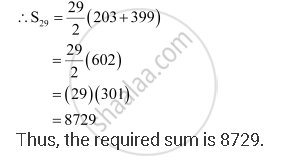Share

# Find the Sum of All Numbers Between 200 and 400 Which Are Divisible by 7. - Mathematics

#### Question

Find the sum of all numbers between 200 and 400 which are divisible by 7.

#### Solution

The numbers lying between 200 and 400, which are divisible by 7, are

203, 210, 217, ­­­­­­­­… 399

∴First term, a = 203

Last term, l = 399

Common difference, d = 7

Let the number of terms of the A.P. be n.

∴ an = 399 = a + (n –1) d

⇒ 399 = 203 + (n –1) 7

⇒ 7 (n –1) = 196

⇒ n –1 = 28

⇒ n = 29Is there an error in this question or solution?